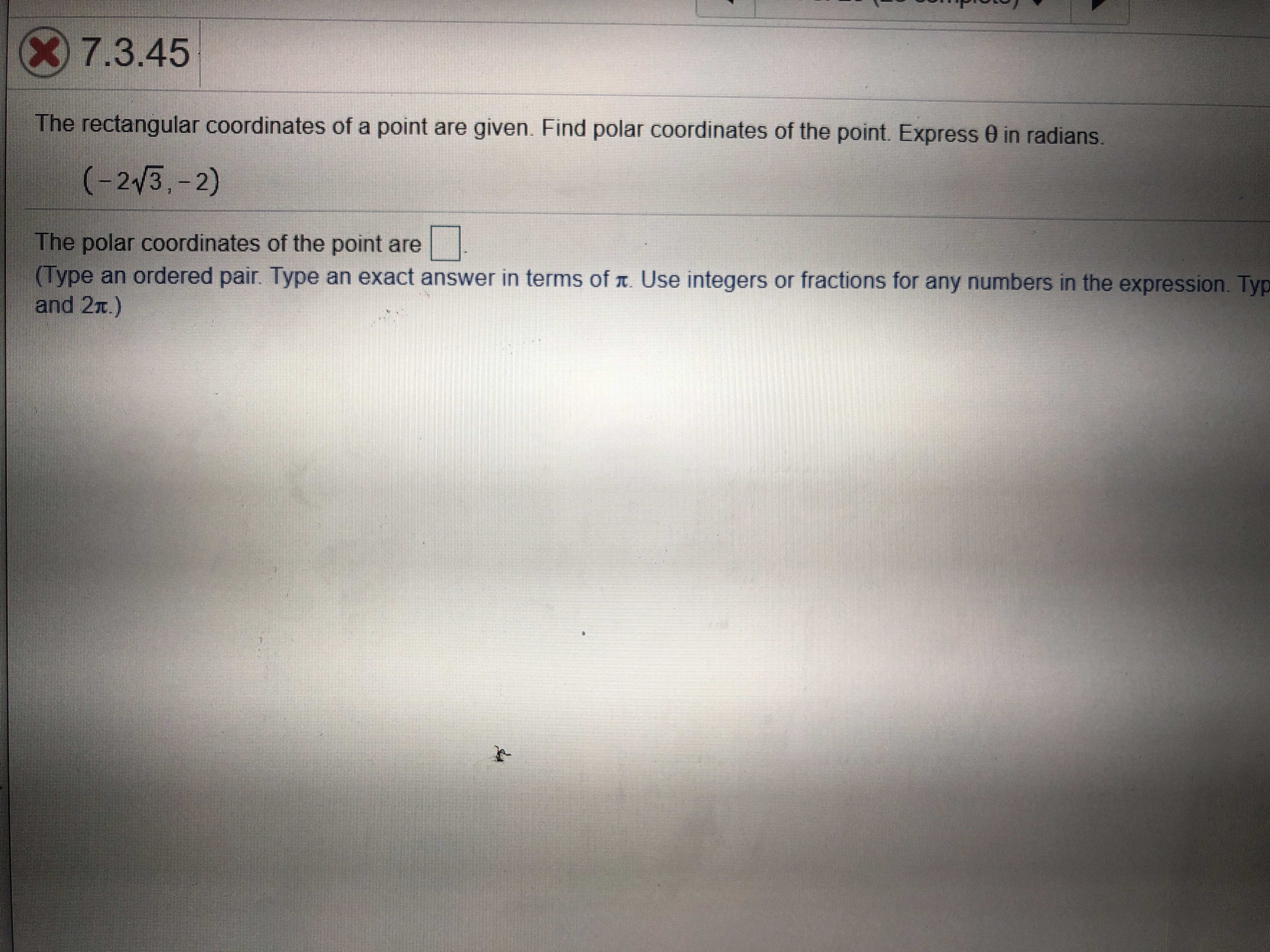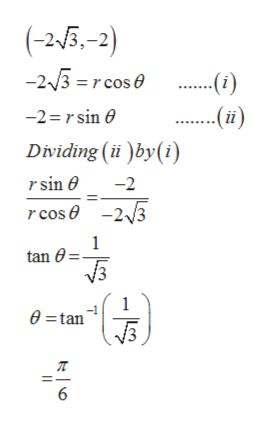# 7.3.45The rectangular coordinates of a point are given. Find polar coordinates of the point. Express 0 in radians.(-23,-2)ww.w.The polar coordinates of the point are(Type an ordered pair. Type an exact answer in terms of . Use integers or fractions for any numbers in the expression. Typand 2T.)

Question
6 views

Can you help with this?help_outlineImage Transcriptionclose7.3.45 The rectangular coordinates of a point are given. Find polar coordinates of the point. Express 0 in radians. (-23,-2) ww.w. The polar coordinates of the point are (Type an ordered pair. Type an exact answer in terms of . Use integers or fractions for any numbers in the expression. Typ and 2T.) fullscreen
check_circle

Step 1

The rectangular coordinates of a point are given, Find the polar coordinates of the point.

Step 2

Let x= r cosθ, y = r sinθhelp_outlineImage Transcriptionclose(-25-2) -23 rcos -2 rsin Dividing ( )by(i) r sin -2 cos -23 1 tan 0 0=tan 6 fullscreen
Step 3

Plugging θ in equa...

### Want to see the full answer?

See Solution

#### Want to see this answer and more?

Solutions are written by subject experts who are available 24/7. Questions are typically answered within 1 hour.*

See Solution
*Response times may vary by subject and question.
Tagged in

### Other